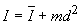Equation Sheet for Engineering Statics

Moment of a force: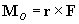Scalar projection of  A along e: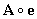Equivalent systems: Two systems are equivalent if the resultant force for the systems is equal and if the resultant moment for the systems is equal

Equilibrium for a particle: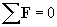Equilibrium for a rigid body: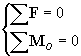Centroid of a line: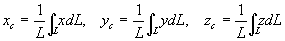Composite bodies: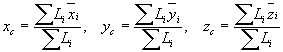Centroid of an area: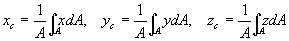Composite bodies:Centroid of a volume: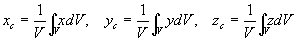Composite bodies: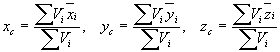Theorems of Pappus:

Surface of revolution: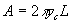Volume of revolution: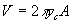Static friction: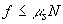,    Pending Motion: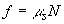Kinetic friction: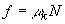Belt friction:Area moment of inertia:,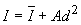,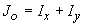Mass moment of Inertia:,